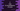# 3 ways to find the area and circumference of a circle in C## How to find the area and circumference of a circle in C:

Let’s learn how to find the area and circumference of a circle in C programming language in this post. We need the radius of a circle to find the area and circumference of a circle. If the radius is r, we can use the below formula to find the area and circumference:

Area: `π * r * r`

Circumference: `2 * π * r`

Where, π is the mathematical constant PI, which is equal to 3.14159 approximately.

So, if we can have the radius as input from the user, we can calculate the area and circumference of that circle.

### Algorithm to find the area and circumference of a circle:

As mentioned before, we need the radius of a circle to calculate its area and circumference. We can take the value of the radius as an input from the user to calculate these values. The C program will use the below algorithm:

• Take the radius as an input from the user.
• Create a constant value to hold the value of PI
• Calculate the area by using the formula π * r * r. Store the calculated value in a variable.
• Calculate the circumference with the formula 2 * π * r. Store it in another variable.
• Print the calculated area and circumference.

### Method 1: C program to find the area and circumference of a circle with user input radius:

Let’s write down the C program by using the above formula:

``````#include <stdio.h>

int main()
{
double PI = 3.14159;
double area, circumference;

printf("Enter the value of the radius: ");

circumference = 2 * PI * radius;

printf("Area: %lf, Circumference: %lf\n", area, circumference);
}``````

Here,

• radius is a double variable to hold the radius of the circle. PI is used to hold the value of π. area and circumference variables are used to hold the area and circumference of the circle.
• It asks the user to enter the value of the radius. It reads the value and stores it in the radius variable.
• The area and circumference are calculated by using the formulae we discussed above. The last line is printing the calculated area and circumference values.

If you run this program, it will print the output as below:

``````Enter the value of the radius: 14
Area: 615.751640, Circumference: 87.964520``````

### Method 2: C program to find the area and circumference of a circle by using a separate function:

We can use two separate functions to find the area and circumference. These functions will take the radius of the circle as the parameter and return the area or circumference. If we use a separate function, we can call it from various places in our program. Let’s re-write the above program to use separate functions to calculate area and circumference:

``````#include <stdio.h>
#define PI 3.14159

{
}

{
return 2 * PI * radius;
}

int main()
{
double area, circumference;

printf("Enter the value of the radius: ");

printf("Area: %lf, Circumference: %lf\n", area, circumference);
}``````

We made two changes to this program.

• We introduced a macro to hold the value of π.
• We created two new functions to calculate the area and circumference of a circle.
• The getArea function is used to calculate and get the area of a circle and the getCircumference function is used to calculate and get the circumference of a circle. Both of these functions takes the radius as the parameter and return the calculated values.
• These methods are called to calculate the area and circumference in the main method.

It will give a similar result.

``````Enter the value of the radius: 12
Area: 452.388960, Circumference: 75.398160``````

### Method 3: C program to find the area and circumference of a circle by using predefined PI constant:

In the above examples, we are creating a constant to hold the value of PI. We can also use the predefined constant value of PI. This value is already defined in math.h header file and we can use the M_PI constant to use the value of Pi.

Let’s rewrite the above program to use M_PI as the PI value:

``````#include <stdio.h>
#include <math.h>

{
}

{
return 2 * M_PI * radius;
}

int main()
{
double area, circumference;

printf("Enter the value of the radius: ");
``````Enter the value of the radius: 12